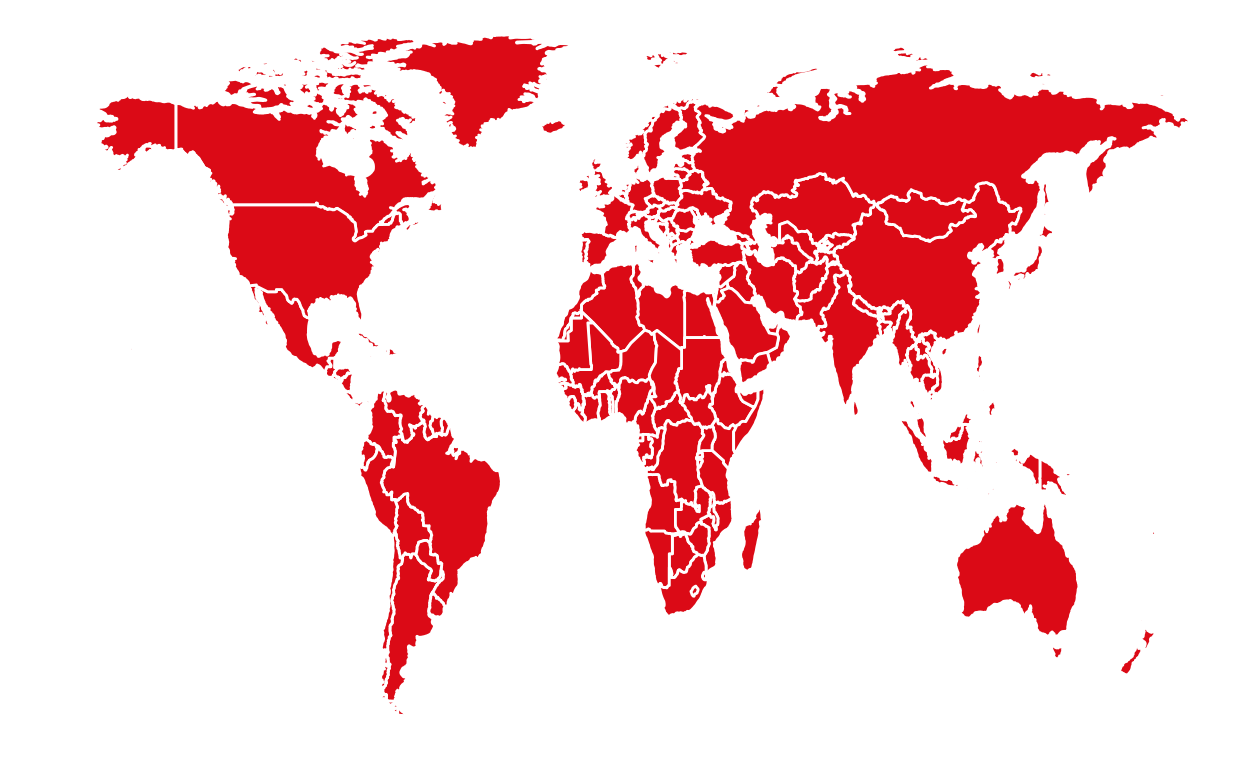# [API] Quandl

Access financial and economic data through the Quandl API.

Thierry Warin https://warin.ca/aboutme.html (HEC Montréal and CIRANO (Canada))https://www.hec.ca/en/profs/thierry.warin.html
06-01-2020## Database description

Quandl is a data agregator. It collects and shares business data with multiple sources, including financial, economics and alternatives datasets.

Quandl : https://www.quandl.com/

### API key

To access Quandl data, you need an API key that is provided by the institution. First, you have to (freely) register to the institution at: https://www.quandl.com/sign-up-modal?defaultModal=showSignUp&intendedUrl=%2Faccount%2Fprofile. After creating an account, you will be provide with a unique API key. Such key will be in the form of:

APIkey <- “abcdefghijklmnopqrstuvwxyz123456”

After confirming your email adress to validate the key, you will have to enter your API key in the function Quandl.api_key(). This will take the API Key given by Quandl and will allow the access to the database.

``````# Register API key
library(Quandl)
Quandl.api_key("abcdefghijklmnopqrstuvwxyz123456")
``````

Now, with your API key you can access data sources of Quandl

This library gives access to financial and economic datasets from hundreds of publishers on one convenient platform

## Functions

Quandl supports two data formats: time-series and “datatables” (used for non-time-series data). You have to look each data product’s documentation to determine which format it employs. Then, use the appropriate calls as follows:

• Quandl()

• Quandl.datatable()

Each of these functions are detailed in this course and some examples are provided.

### Quandl()

The function Quandl() takes as an input the datasets and the name of the desired indicator. It will return the data from the indicator in a data frame.

This function is used for time series data product (only contain sorted numeric values).

For example, we would like to obtain the US GDP from FRED (Federal Reserve Economic Data).

``````#US GDP from FRED
mydata = Quandl("FRED/GDP")
``````

Filters can be added to target certain data.

In the following example, we would like to know the US GDP between 2002 and 2006.

``````mydata = Quandl("FRED/GDP", start_date="2001-12-31", end_date="2005-12-31")
``````

### Quandl.datatable()

The function Quandl.datatable() takes as an input the datasets and the name of a ticker. It will return the data from the indicator in a data frame.

This function is used for datatables (used for non-time-series data) data product. It can include various unsorted data types :strings, numbers, dates, etc..

For example we would like to retrieve all rows for ZACKS/FC where ticker=‘AAPL’ (Apple).

``````mydata = Quandl.datatable("ZACKS/FC", ticker="AAPL")
``````

Filters can also be added to target certain data for the datatables data.

In the following example, we would like to know the total revenues of Apple and Microsoft before 2015.

``````mydata = Quandl.datatable("ZACKS/FC", ticker=c("AAPL", "MSFT"), per_end_date.gt="2015-01-01",qopts.columns=c("m_ticker", "per_end_date", "tot_revnu"))
``````

## tl;dr

``````#Loading the Quandl library
library(Quandl)
#US GDP from FRED (time series data)
mydata = Quandl("FRED/GDP")
#specific data:
mydata1 = Quandl("FRED/GDP", start_date="2001-12-31", end_date="2005-12-31")
#ZACKS/FC where ticker='AAPL' (datatables data)
mydata2 = Quandl.datatable("ZACKS/FC", ticker="AAPL")
#specific data:
mydata3 = Quandl.datatable("ZACKS/FC", ticker=c("AAPL", "MSFT"), per_end_date.gt="2015-01-01",qopts.columns=c("m_ticker", "per_end_date", "tot_revnu"))
``````

## Code learned this week

Command Detail
Quandl() Find data from datasets in the time series format
Quandl.datatable()() Find data from datasets in the “datatables” format

This tutorial uses the Quandl documentation